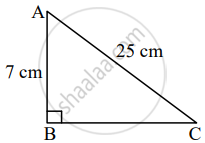# In a right angled triangle, if length of hypotenuse is 25 cm and height is 7 cm, then what is the length of its base? - Geometry

Sum

In a right angled triangle, if length of hypotenuse is 25 cm and height is 7 cm, then what is the length of its base?

#### SolutionLet ∆ABC be the given right angled triangle.

AC = 25 cm, AB = 7 cm

In ∆ABC, ∠B = 90°     ......[Given]

∴ AC2 = AB2 + BC2    .......[Pythagoras theorem]

∴ 252 = 72 + BC2

∴ 625 = 49 + BC2

∴ BC2 = 625 – 49

∴ BC2 = 576

∴ BC = 24 cm    .......[Taking square root of both sides]

∴ The length of the base of the given right angle triangle is 24 cm.

Concept: Right-angled Triangles and Pythagoras Property
Is there an error in this question or solution?

Share